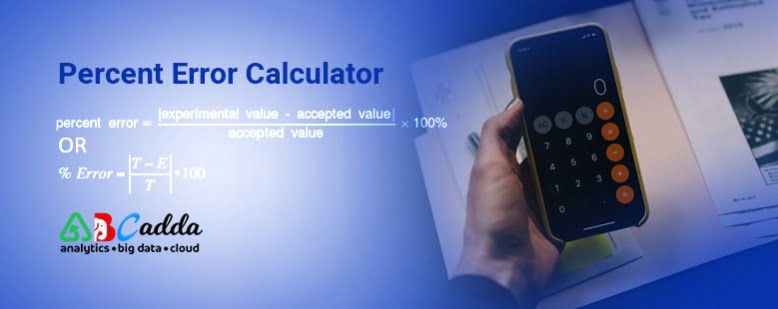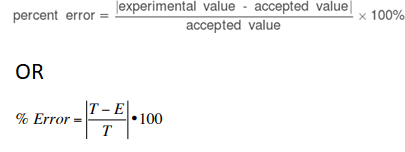# Percentage/Percent Error Calculator | Percent error formulaThis percentage/percent error calculator calculates the percentage error between the experimentally measured value and the theoretical actual value. Percent Error Calculator is a free online tool that shows the percentage of errors / percentage erroe. Our online percent error counting tool speeds up calculations and displays the percentage of errors in a fraction of a second.

To use a percent error rate calculator, first enter the true values of everything you have measured. Include any assumptions, observations, measurements, or values in measured values, modeled in units that are actually the same. Then click the Calculate button and we will calculate the error rate.

## Percent error calculator | Percentage error calculator

Accepted (True Value)
Percent Error
%

The percent error shows how much we did wrong when we measured something in the analysis process. A smaller error rate indicates that we are closer to the accepted or initial value. For instance, a 2% percent error demonstrates that we are extremely near the obtained value, while 48% implies that we are very far from the true value.

Measurement errors are often unavoidable for some reason, such as, the hands are shaking, the material is incorrect or our instrument cannot estimate accurately. The percent error formula provides insight into how strongly this inevitable error affects our results.

This percent error calculator determines the relative error between the true value and the observed value found during measurement. Thanks to this tool, you can quickly judge whether your measurements are correct.

Read on to learn how to calculate the percent error rate and find the percent error formula. You can also look at the percent calculator to better understand the concept of percentages.

Let’s use this percent error calculation to find the difference between your estimate and the actual value. We often prefer to count values rather than count them all the time.

We have developed this error rate calculator for calculating percent error which will make your calculations and estimates perfect. This percentage error calculator is useful when you have used estimated values in complex calculations.

The percentage error value is very important in experimental calculations. This allows us to see how far along our estimate is and the exact value as a percentage. We therefore need to calculate the error rate in many situations.

In this topic we will discuss what is percent error and the percent error rate formula with examples.

## What is Percent Error Calculator | percentage error calculator

Percentage error, also known as percent error, is an expression of the difference between a measured value and a known or accepted value. It is widely used in science to explain the difference between experimental value and expected value.

It is basically an error that is calculated to determine the difference between two values known as the actual value and the observed value. Any measurement error can lead to false or negative results that everyone wants to avoid.

One of the main measurement errors is percentage error. You can calculate the percentage error using the percentage error calculator. In principle it represents the error between the value received and the value observed.

As long as the value measurement study is in progress, the results may differ from the values received. The reasons for this measurement error may vary. This could be due to a major reason such as equipment malfunction or human error. This is the most widely recognized explanation behind the percent error.

In this scenario, it’s a good idea to calculate the mistakes to avoid.

### Important Points about Percent Error Calculator | percent error formula

• The purpose of calculating the percentage error is to estimate how close the measured value is to the true value.
• In some fields, percentage error is always represented as a positive number. In other cases, it is true if it has a positive or negative value. The mark can be stored to determine whether the recorded value is consistently above or below the expected value.
• Percentage error is a kind of error calculation whereas Absolute and relative error calculator are two other common calculations. Error rates are part of a comprehensive error analysis.
• The key to reporting the error rate correctly is knowing whether to include a sign (positive or negative) in the calculation and reading the value with the correct number of significant digits.

## How to calculate percent error by using Percent error formula

Percent error is the difference between the measured or experimental value and the accepted or known value divided by the known value multiplied by 100%. In many applications, the percentage of error is always expressed as a positive value.

The absolute value of the error is divided by the value received and expressed as a percentage.

It is common for chemistry and other sciences to maintain negative values when they exist. Whether the error is positive or negative is important.

For example, you would not expect a positive error rate when comparing actual versus theoretical yields of chemical reactions. If a positive value is calculated, it will indicate a possible problem with the procedure or a reaction that was not reported.

If the error sign is retained, the calculation is the experimental or measured value minus the known or theoretical value divided by the theoretical value and multiplied by 100%.

### Percent error equation | can percent error be negative

Percent Error rate = [experimental value – theoretical value] / theoretical value x 100%
The formula for percent error is:Here,
T = True or Actual value
E = Estimated value

Note: Sometimes it is helpful to know if an error is positive or negative. If you need to know false positives or negatives, insert absolute brackets into the formula to do it. In most cases, absolute error is true. For example, experimenting with the results of chemical reactions is unlikely to give you more than is theoretically possible.

## Percent error calculator formula

It’s about how the error percentage is calculated manually. We are pleased that you are offered a calculating percent error whose calculations can be performed very easily and quickly. Now you don’t have to worry about solving the equation to get the result. You can enjoy this in no time. You need to have data that can be entered into the calculator.

Calculating the percentage error is very easy with the percentage error calculation. The error in the target percentage is very fast if you enter the value in the given slot and then press the calculate button.

The error percentage is calculated automatically with the percentage error calculator and the result is displayed on the screen.

### Steps for Calculating Percentage Errors by using percent error equation

• Subtract the received value from the experimental value.
• Take the absolute value from step 1
• Multiply the answer by 100 and add a% symbol to express the answer as a percentage.

### How to find percent error let’s try an example

You will receive a cube of pure honey. Measure the sides of the cube for volume and weigh it for mass. If you calculate the density from your measurement, you get 8.78 grams / cm3. Accepted copper density is 8.96 g / cm³. What is your percentage error?

## How to calculate percentage error solution

```Follow the formula for percent error
Experimental value = 8.78 g / cm³
Theoretical value = 8.96 g / cm3
```

Step 1: Subtract the assumed value from the experimental value

`8.78 g / cm³ - 8.96 g / cm³ = -0.18 g / cm³`

Step 2: take the absolute value from step 1

`| -0.18 g / cm³ = 0.18 g / cm³`

`0.18 g / cm³/ 8.96 g / cm³=0.02`

Step 4: Multiply the answer by 100 and add a% symbol to express the answer as a percentage

```0.02 x 100 = 2
2%
The percentage error in calculating your density is 2%.
```

## How to find percent error 2nd example | calculating percent error

You will receive an aluminum block in the laboratory. Measure the dimensions of the blocks and their displacement on a ship with a given volume of water. Calculate the density of the aluminum block to be 2.10 g / cm3. Find the density of an aluminum block at room temperature and find that it is 1.12 g / cm3. Calculate the percentage error of your measurement.

### How to calculate percentage error for the above example Solution | percent error equation

• Subtract one value from the other: 2.10 – 1.12 = -0.98
• Depending on what you need, you can discard any negative sign (take the absolute value): 0.98 This is the error.
• Divide the error by the true value: 0.02 / 2.70 = 0.875
• Multiply this value by 100% to get the error percentage
• 0.875 x 100% = 87.50% (expressed as 2 significant numbers). Numbers are of significant importance in science. Reporting too many or too few answers can be considered wrong even if you set the problem correctly.

## Percent Error rate, margin of error, and standard error

There are many terms which are similar to error rate. In order not to confuse and confuse them, here is a brief explanation of each. The percentage error or relative error calculator is exactly what we described above – the relative difference between the observed value and the true value.

You can use the percentage error formula above to calculate it. Standard error is a computed value for a data set. This is a statistical term that describes the accuracy of a sample representing an entire population.

In contrast, the margin of error is closely related to the confidence interval. This can be calculated by multiplying the standard error by the z-score, which corresponds to a certain confidence level.

#### Conclusion

Mathematicians and scientists alike want to know whether theoretical ideas are closer to actual results. You can utilize the error percent rate to decide the connection between what really occurred and what you anticipated.

The basic assumption for finding the percentage of error is that the subject has real value. He must be aware of the value received or correct in order to continue. However, there are cases when the true value is unknown.

All things considered, for this situation, you don’t need to worry by any means. However, you have the option to calculate the error rate. Then how do you calculate% error?

Well, in a scenario like this, standard deviation can help you when real values are rejected. This makes it easier for the math to represent errors